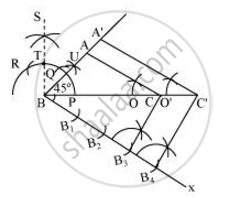# Draw a triangle ABC with side BC = 7 cm, ∠B = 45°, ∠A = 105°. Then, construct a triangle whose sides are 4/3 times the corresponding side of ΔABC. Give the justification of the construction. - Mathematics

Draw a triangle ABC with side BC = 7 cm, ∠B = 45°, ∠A = 105°. Then, construct a triangle whose sides are 4/3 times the corresponding side of ΔABC. Give the justification of the construction.

#### Solution

∠B = 45°, ∠A = 105°

Sum of all interior angles in a triangle is 180°.

∠A + ∠B + ∠C = 180°

105° + 45° + ∠C = 180°

∠C = 180° − 150°

∠C = 30°

The required triangle can be drawn as follows.

Step 1

Draw a ΔABC with side BC = 7 cm, ∠B = 45°, ∠C = 30°.

Step 2

Draw a ray BX making an acute angle with BC on the opposite side of vertex A.

Step 3

Locate 4 points (as 4 is greater in 4 and 3), B1, B2, B3, B4, on BX.

Step 4

Join B3C. Draw a line through B4 parallel to B3C intersecting extended BC at C'.

Step 5

Through C', draw a line parallel to AC intersecting extended line segment at C'. ΔA'BC' is the required triangle.Justification

The construction can be justified by proving that

A'B = 4/3 AB, BC' = 4/3BC , A'C' = 4/3 AC

n ΔABC and ΔA'BC',

∠ABC = ∠A'BC' (Common)

∠ACB = ∠A'C'B (Corresponding angles)

∴ ΔABC ∼ ΔA'BC' (AA similarity criterion)

=>(AB)/(A'B) = (BC)/(BC') = (AC)/(A'C') ....1

In ΔBB3C and ΔBB4C',

∠B3BC = ∠B4BC' (Common)

∠BB3C = ∠BB4C' (Corresponding angles)

∴ ΔBB3C ∼ ΔBB4C' (AA similarity criterion)

=>(BC)/(BC') =

=>(BC)/(BC') = 3/4   ...(2)

On comparing equations (1) and (2), we obtain

(AB)/(A'B) = (BC)/(BC')=(AC)/(A'C') = 3/4

=> A'B = 4/3 AB, BC' = 4/3 BC, A'C' = 4/3 AC

This justifies the construction.

Concept: Division of a Line Segment
Is there an error in this question or solution?
Chapter 11: Constructions - Exercise 11.1 [Page 220]

#### APPEARS IN

NCERT Class 10 Maths
Chapter 11 Constructions
Exercise 11.1 | Q 5 | Page 220

Share